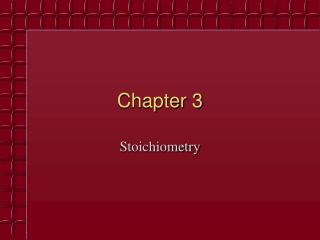Download PresentationChapter 3

# Chapter 3

Download Presentation## Chapter 3

- - - - - - - - - - - - - - - - - - - - - - - - - - - E N D - - - - - - - - - - - - - - - - - - - - - - - - - - -
##### Presentation Transcript

1. Chapter 3 Stoichiometry

2. Chemical Stoichiometry • Stoichiometry - The study of quantities of materials consumed and produced in chemical reactions.

3. Atomic Masses • Elements occur in nature as mixtures of isotopes • Carbon = 98.89% 12C • 1.11% 13C • <0.01% 14C • Carbon atomic mass = 12.01 amu

4. The Mole • The number equal to the number of carbon atoms in exactly 12 grams of pure 12C. • 1 mole of anything = 6.022  1023units of that thing

5. Avogadro’s number equals6.022  1023 units

7. Molar Mass • A substance’s molar mass (molecular weight) is the mass in grams of one mole of the compound. • CO2 = 44.01 grams per mole

8. Determine the formula weights of each of the following compounds: (a) N2O5, (b) CuSO4, (c) (NH4)3PO4, (d) Ca(HCO3)2, (e) aluminum sulfide, (f) iron(III) sulfate, (g) disilicon hexabromide.

9. Calculate the following quantities: (a) mass, in grams, of 1.906 × 10−2 mol Bal2

10. (b) number of moles of NH4Cl in 48.3 g of this substance

11. c) number of molecules in 0.05752 mol HCHO2

12. (d) number of O atoms in 4.88 × 10−3 mol Al(NO3)3

13. The molecular formula of allicin, the compound responsible for the characteristic smell of garlic, is C6H10OS2. (a) What is the molar mass of allicin? (b) How many moles of allicin are present in 5.00 mg of this substance? (c) How many molecules of allicin are in 5.00 mg of this substance? (d) How many S atoms are present in 5.00 mg of allicin?

14. Percent Composition • Mass percent of an element: • For iron in iron (III) oxide, (Fe2O3)

15. Formulas • molecular formula = (empirical formula)n [n = integer] • molecular formula = C6H6 = (CH)6 • empirical formula = CH

16. Empirical Formula Determination • 1. Base calculation on 100 grams of compound. • 2. Determine moles of each element in 100 grams of compound. • 3. Divide each value of moles by the smallest of the values. • 4. Multiply each number by an integer to obtain all whole numbers.

17. Glycine, an amino acid used by organisms to make proteins, is represented by the molecular model below. (a) Write its molecular formula. (b) Determine its molecular mass. (c) Calculate the percent nitrogen by mass in glycine.]

18. Chemical Equations Chemical change involves a reorganization of the atoms in one or more substances.

19. Chemical Equation • A representation of a chemical reaction: • C2H5OH + 3O22CO2 + 3H2O • reactants products

20. Chemical Equation • C2H5OH +3O22CO2+3H2O • The equation is balanced. • 1 mole of ethanol reacts with 3 moles of oxygen • to produce • 2 moles of carbon dioxide and 3 moles of water

21. Calculating Masses of Reactants and Products • 1. Balance the equation. • 2. Convert mass to moles. • 3. Set up mole ratios. • 4. Use mole ratios to calculate moles of desired substituent. • 5. Convert moles to grams, if necessary.

22. Consider the Combustion of Propane (C3H8) • If 5.0 g of propane is burned in excess oxygen: • 1. What mass of CO2 is produced? • 2. How many grams of O2 are needed for complete combustion of the propane? Movie

23. Calcium hydride reacts with water to produce calcium hydroxide and hydrogen gas. • A. Write a balanced chemical equation for the reaction. • B. How many grams of calcium hydride are needed to produce 5.0 g of hydrogen gas.

24. Limiting Reactant The limiting reactant is the reactant that is consumed first,limiting the amounts of products formed. Movie

25. Solving a Stoichiometry Problem • 1. Balance the equation. • 2. Convert masses to moles. • 3. Determine which reactant is limiting. • 4. Use moles of limiting reactant and mole ratios to find moles of desired product. • 5. Convert from moles to grams.

26. 2CH3OH +3O22CO2+4H2O Given 1.0 g of CH3OH and 1.0 g of O2, how much CO2 will be produced in this combustion reaction?

27. END